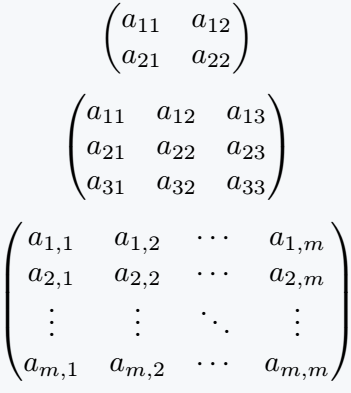# What is pmatrix in LaTeX?

Latex environment is a logical structural process in which various functional tasks are completed by passing arguments and parameters.

This LaTeX environment is bound by two commands. That is, it starts with \begin{arg} command and ends with \end{arg} command.

\begin{arg}
..............
..............
\end{arg}

And the same argument is passed between the two commands, which indicates what kind of task the environment will perform!

For example, pmatrix is ​​an argument that returns matrix when passed in this latex environment and whose elements are bound by parenthesis.## Why p is added before matrix?

There are more than one argument for defining different types of matrix. Such as matrix, pmatrix, bmatrix, vmatrix, and Vmatrix, etc.

Elements of the matrix will be bound by parenthesis, for this reason, pmatrix argument has been created by adding p before the matrix.

\documentclass{article}
\usepackage{amsmath}
\begin{document}
$$\begin{pmatrix} a_{11} & a_{12}\\ a_{21} & a_{22} \end{pmatrix}$$
$$\begin{pmatrix} a_{11} & a_{12} & a_{13}\\ a_{21} & a_{22} & a_{23}\\ a_{31} & a_{32} & a_{33} \end{pmatrix}$$
$$\begin{pmatrix} a_{1,1} & a_{1,2} & \cdots & a_{1,m} \\ a_{2,1} & a_{2,2} & \cdots & a_{2,m} \\ \vdots & \vdots & \ddots & \vdots \\ a_{m,1} & a_{m,2} & \cdots & a_{m,m} \end{pmatrix}$$
\end{document}

Output :#### Md Jidan Mondal

LaTeX expert with over 10 years of experience in document preparation and typesetting. Specializes in creating professional documents, reports, and presentations using LaTeX.